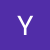# Outline:

1. Definition of the Image Segmentation
2. What is K-Means?
3. How to perform the image segmentation with the K-Means clustering algorithm?

# Fourier Series and Fourier Transform. Signal Denoising.

1. Fourier Series
2. Fourier Transform
3. Signal Denoising using the Fourier transform

# Outline:

1. Some New Terminologies
2. Euler, Hamiltonian, and Bipartite graphs
4. Depth-First Search

# Some New Terminologies

1. The null Graph does not contain any edge. There is no connection between the vertices.

# Derivation of the Normal Equation of Linear Regression problem